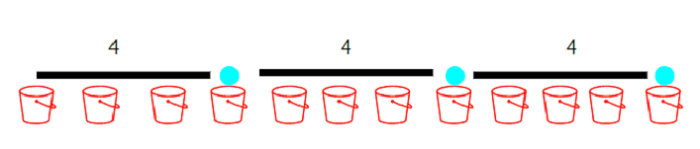# Program to maximize the minimum force between balls in a bucket using Python

Suppose we are given several buckets and x number of balls. If the balls are put into the bucket, a special force acts within them and we have to find out a way to maximize the minimum force between two balls. The force between two balls in a bucket of position p and q is |p - q|. The input given to us is the array containing the bucket positions and the number of balls x. We have to find out the minimum force between them.

So, if the input is like pos = [2, 4, 6, 8, 10, 12], x = 3, then the output will be 4.The balls can be put into the given positions in an array of 12 buckets. The three balls can be put into positions 4, 8, and 12 and the power between them will be 4. This value cannot be increased further.

To solve this, we will follow these steps −

• Define a function ball_count() . This will take d

• and := 1

• curr := pos

• for i in range 1 to n, do

• if pos[i] - curr >= d, then

• ans := ans + 1

• curr := pos[i]

• return ans

• n := size of pos

• sort the list pos

• left := 0

• right := pos[-1] - pos

• while left < right, do

• mid := right - floor value of((right - left) / 2)

• if ball_count(mid) >= x, then

• left := mid

• otherwise,

• right := mid - 1

• return left

## Example

Let us see the following implementation to get better understanding −

def solve(pos, x):
n = len(pos)
pos.sort()

def ball_count(d):
ans, curr = 1, pos
for i in range(1, n):
if pos[i] - curr >= d:
ans += 1
curr = pos[i]
return ans

left, right = 0, pos[-1] - pos
while left < right:
mid = right - (right - left) // 2
if ball_count(mid) >= x:
left = mid
else:
right = mid - 1
return left

print(solve([2, 4, 6, 8, 10, 12], 3))

## Input

[2, 4, 6, 8, 10, 12], 3

## Output

4